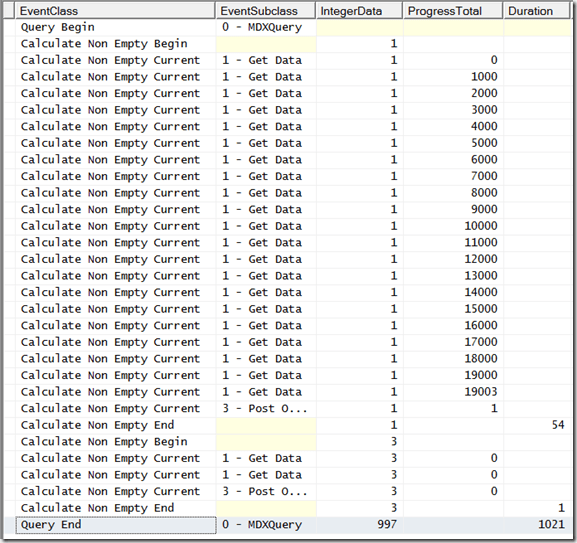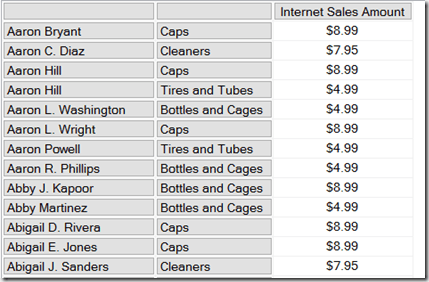# Monitoring SSAS Multidimensional Non Empty Filtering Using Profiler, Part 3

In Part 1 of this series I introduced the different types of non empty filtering that occur in Analysis Services Multidimensional and in Part 2 I showed how you can use monitor this activity using Profiler. In this, the final part of the series, I’m going to show some examples of how you can use this information while tuning MDX queries.

Let’s start by looking at the following query:

```SELECT
{[Measures].[Internet Sales Amount]}
ON 0,
NON EMPTY
[Customer].[Customer].[Customer].MEMBERS
*
[Product].[Subcategory].[Subcategory].MEMBERS
ON 1
FROM
WHERE([Date].[Calendar Year].&)
```

It returns 19004 rows – all of the combinations of Customer and Subcategory that have a value in the year 2003:Here’s what you can see in Profiler:There are two Non Empty operations here: the ProgressTotal column shows that first is the NON EMPTY statement on the rows axis; the second we can ignore because it’s the evaluation of the WHERE clause. The Duration column shows that the first Non Empty operation takes just 54ms and the query as a whole takes 1021ms.

Now, let’s make things a bit more complicated by adding an extra filter so we only see the Customer/Subcategory combinations where Internet Sales Amount is less than \$10:

```SELECT
{[Measures].[Internet Sales Amount]}
ON 0,
NON EMPTY
FILTER(
[Customer].[Customer].[Customer].MEMBERS
*
[Product].[Subcategory].[Subcategory].MEMBERS
,
[Measures].[Internet Sales Amount]<10)
ON 1
FROM
WHERE([Date].[Calendar Year].&)
```Here’s what Profiler shows:The query now takes 2512ms. But why is it slower? The obvious assumption to make is that it’s the Filter() that has slowed things down, but it looks like the Filter() and the NON EMPTY are now being evaluated as a single operation because the first Non Empty operation in the trace is now taking 2408ms – the majority of the query duration.

Removing the NON EMPTY statement from the rows axis and putting the logic to filter out the customers with no sales into the Filter() function, like so:

```SELECT
{[Measures].[Internet Sales Amount]}
ON 0,
FILTER(
[Customer].[Customer].[Customer].MEMBERS
*
[Product].[Subcategory].[Subcategory].MEMBERS
,
[Measures].[Internet Sales Amount]<10
AND
(NOT ISEMPTY([Measures].[Internet Sales Amount])))
ON 1
FROM
WHERE([Date].[Calendar Year].&)
```

…only makes things worse, increasing query duration to 4139ms. This confirms our suspicion that Filter() is the problem here and that NON EMPTY can remove the empty customers faster than Filter() can.

The problem with the last query but one is that the NON EMPTY statement is being applied after the Filter(). Wouldn’t it be faster to remove the empty customers first and then filter out the ones where Internet Sales Amount is less than \$10, so the slower Filter() can be applied over a smaller set?

There are two ways we can do this. First of all, we can use the NonEmpty() function instead of the NON EMPTY statement to remove the empty customers. NonEmpty() is not faster than the NON EMPTY statement per se, but it does allow us to change the order that the different types of filtering are applied here, and that can make all the difference to performance. Here’s a new version of the query:

```SELECT
{[Measures].[Internet Sales Amount]}
ON 0,
FILTER(
NONEMPTY(
[Customer].[Customer].[Customer].MEMBERS
*
[Product].[Subcategory].[Subcategory].MEMBERS,
[Measures].[Internet Sales Amount]),
[Measures].[Internet Sales Amount]<10)
ON 1
FROM
WHERE([Date].[Calendar Year].&)
```Query duration is now down to 217ms and the first Non Empty operation is only 57ms.

There’s another way of doing this. For MDX geek-points you could use the ultra-obscure HAVING clause in your query to do the filtering after the NON EMPTY, like so:

```SELECT
{[Measures].[Internet Sales Amount]}
ON 0,
NON EMPTY
[Customer].[Customer].[Customer].MEMBERS
*
[Product].[Subcategory].[Subcategory].MEMBERS
HAVING
[Measures].[Internet Sales Amount]<1000
ON 1
FROM